telescopeѲptics.net          ▪▪▪▪                                             CONTENTS

8.2.4. DALL-KIRKHAM TELESCOPE

An interesting two-mirror variant is one with the secondary left spherical. It is known as Dall-Kirkham and, although with stronger coma than its classical counterparts, its mirrors are easier to make, and its miscollimation sensitivity is considerably lower than in either classical or aplanatic Cassegrain, which makes it a viable practical alternative. With the secondary spherical, needed primary conic for corrected Seidel spherical aberration, from Eq. 80, iswhere m, η and k are the secondary magnification, back focus (primary-to-final-focus separation) in units of primary's focal length and relative height of marginal ray at the secondary (i.e. minimum secondary radius) in units of the aperture radius, respectively.

In the configuration with convex secondary, called Dall-Kirkham Cassegrain, the primary is ellipsoidal (it is hyperboloidal with concave secondary, which makes such arrangement impractical). The system coma wavefront error in Dall-Kirkham configuration - and in a general two-mirror system with spherical secondary - is larger by a factor 1+[(m2-1)(m-η)/2m], than in a comparable classical Cassegrain.

The astigmatism, however, is different by a factor 1-[(m2-1)(m-η)2/4m(m2+η)] which, considering the sign of m, means it is smaller in Dall-Kirkham, but larger in the Gregorian with spherical secondary. As a result, the DK median field surface is less curved, as given by: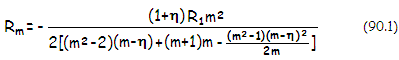and so is median surface in the Gregorian, since its astigmatism - unlike that in the DK - makes it less curved than its Petzval curvature (median surface curvature in the Gregorian is approximately inversely proportional to the level of astigmatism).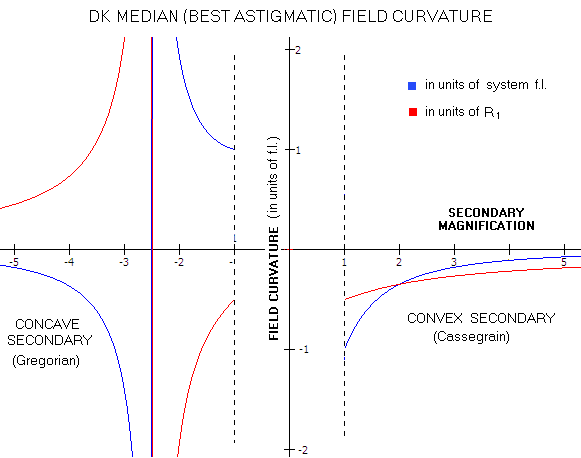The graph shows median field curvature for two mirror system with spherical primary, either convex or concave (based on Eq. 90.1, and η=0.25). The curvature expressed in system focal lengths is of the opposite sign from the actual curvature for the arrangement with concave secondary mirror (Gregorian), which has numerically negative focal length. Best image can be flat only in the Gregorian, with m=-2.5, with the minimum secondary size is k=(1+η)/(m+1)=-0.83. For the practical range of secondary magnifications, about |4| and larger,  the Gregorian has significantly less curved best image.

In addition to being less curved, the DK image is fairly insensitive to defocus error caused by image field curvature, due to the present off-axis coma being significantly larger than the defocus error caused by field curvature. As a result, Dall-Kirkham flat-field performance is very close to its best field performance for small to moderate field sizes.

Note that since the coma wavefront error in a classical Cassegrain is inversely proportional to F2 over angular field, and to F3 over linear field, thus for given primary diminishes with the square of secondary magnification angularly, and with the cube of it linearly, the actual error for a given field angle in the DK is offset by this factor (F, as elsewhere on this site, is the system focal ratio).

Since the angular-field coma in the DK vs. classical Cassegrain for η=0 changes in proportion to (m2+1)/2, while at the same time changing in inverse proportion to F2 (i.e. m2), the two systems with given primary equalize in this respect when [(m2+1)/2](m0/m)2 equals 1, with m0 and m being the classical Cassegrain and DK secondary magnifications, respectively. This implies that secondary magnification in the classical Cassegrain for the angular-field coma nearly equalized is given by m0~2m/(m2+1)1/2, and that DK secondary magnification needs to be m~m0/(2-m02)1/2 for near equal level of angular coma, with m being the classical Cassegrain secondary magnification.

It is obvious that DK can equalize with classical Cassegrain only if the latter has secondary magnification lower than 2. For larger secondary magnification, angular-field coma in the DK will remain larger, approximately in proportion to [(m2+1)/2](m0/m)2.

It is, however, different for coma corrected linear field. Here, the DK can always compensate by increasing secondary magnification. The two arrangements nearly equalize for [(m2+1)/2](m0/m)3=1, with the needed classical Cassegrain's secondary magnification given as m0~[2m3/(m2+1)]1/3. So DK with secondary magnification m=5 will have similar linear field quality as classical Cassegrain with secondary magnification m0=2.1. For an ƒ/4 primary, that would come to ƒ/20 and ƒ/8.4 system for classical and DK Cassegrain, respectively.

Angular coma in the DK is proportional to its coma aberration coefficient,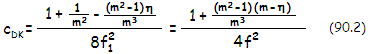with the corresponding best focus P-V wavefront error of coma: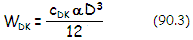This means that, given primary, the DK coma for given field angle weakly decreases with the increase in secondary magnification, while its linear coma-free field increases nearly in proportion to it. Since the second and third factor in the numerator of Eq. 90.2 nearly offset for usual values of m, the P-V wavefront error of a system with spherical secondary, either Cassegrain or Gregorian, is well approximated by WDK~αD3/96ƒ12= αD/96F12, ƒ1 being the primary's focal length.

Aberration coefficient for astigmatism is aDK=[4(m2+η)m-(m2-1)(m-η)2]/8(1+η)ƒm2, with the P-V wavefront error of astigmatism at the best focus WDKa=aDK(αD)2/4.

In general, Cassegrain arrangement with spherical secondary requires about half as aspherised primary as the Gregorian, which is in the latter hyperboloid. Astigmatism in the latter is significantly larger, but it flattens otherwise very strongly curved field, with best image surface likely to be somewhat milder than in a comparable Cassegrain. However, for given primary, the Gregorian also requires nearly doubled secondary magnification for accessible final focus. For identical system focal length with given primary, Gregorian requires secondary larger by ~50% to nearly doubled, for the range of secondary magnification from ~|5| to below |3|, respectively. With focal lengths nearly equal, Gregorian has roughly half the field curvature, but it doesn't translate into advantage for visual observing. Coma is of similar magnitude in both; in the Gregorian with similarly sized secondary and nearly doubled focal length, linear diffraction-limited field is correspondingly larger.

While it is evident that both, secondary magnification and final system focal ratio can be the factors determining level of the DK system coma, it is good to clarify the specifics of it. Secondary magnification is only the factor with a given system focal ratio. In other words, an ƒ/15 DK system will have more coma if the secondary magnification is 5 (with an ƒ/3 primary) than when it is 3 (with an ƒ/5 primary). From the above relation for the DK system coma, good approximation of the ratio of increase of the coma wavefront error in the Dall-Kirkham vs. classical Cassegrain (or paraboloid) of the same focal ratio is obtained after setting back focal length η to zero, as w~(m2+1)/2. For the above example, that gives the coma error @5x secondary magnification some 13 times larger than in a comparable Cassegrain, and larger by a factor of 2.6 than @3x secondary magnification.

However, for any given primary focal ratio, secondary magnification and final system focal ratio are not significant factors in determining the final DK system coma level - as long as we are concentrated on the wavefront error for given field angle (angular field coma). The coma wavefront error at any given field angle changes nearly in proportion to (1+1/m2), thus an ƒ/4/24 DK (m=6) will have it little over 10% smaller than an ƒ/4/12 system (m=3). But at the same time, the former system will have more than twice larger linear coma-free field.

It is convenient to express the DK coma level in terms of coma of the paraboloidal mirror. Knowing that for the factor of change Δw in the coma wavefront error of the DK vs. comparable classical Cassegrain (or paraboloid) of identical ƒ-ratio Δw=wDK/wP, the corresponding effective focal ratio is found from F'A=F/Δw1/2, the focal ratio of a comparable paraboloid with respect to the size of Dall-Kirkham's angular diffraction-limited field is approximated by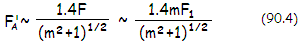F being the DK system focal ratio. The effective focal ratio F'A corresponding to the size of linear diffraction-limited field is found from F'L=F/Δw1/3, and the focal ratio of a comparable paraboloid is approximated by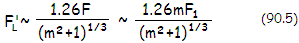Note that simplified form of either of the two approximations - F'A~1.4F1 and F'L~1.26F1m1/3, respectively (F1=F/m=ƒ1/D, the DK primary focal ratio), is still quite useable as an approximation for secondary magnification ~3 and larger. It puts the ƒ/15 DK @3x secondary magnification at the level of an ƒ/6.6 and ƒ/9, while the one @5x secondary magnification at the level of an ƒ/4.2 and ƒ/6.4 paraboloid, for the angular and linear field, respectively. Following diagram shows the relation between relative aperture of the DK primary and the system's diffraction-limited field size in terms of a corresponding paraboloid of focal number F' (the actual system focal ratio F=mF1).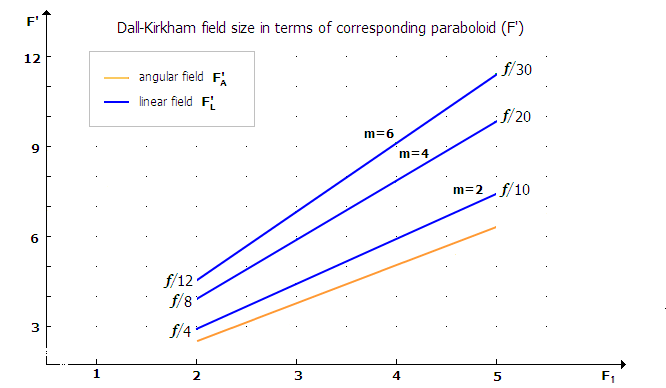FIGURE 123: Diffraction-limited field size in the Dall-Kirkham two-mirror telescope in terms of the field in a paraboloid (F'), DK secondary magnification m, and DK primary mirror focal ratio F1.The paraboloid's focal ratio F'A corresponding to Dall-Kirkham's angular field size is nearly independent of the secondary magnification m, and increases linearly with ~1.4F1. For the linear field size, the corresponding paraboloid's focal ratio F'L also increases linearly, with ~1.26F1, for given secondary magnification, and for given primary it changes nearly with m1/3 for m~3 and larger. Since the quality linear field with coma changes with the third power of the effective focal ratio F'L, it follows that the quality linear field diameter in the Dall-Kirkham with given primary is nearly proportional to its secondary magnification, the higher magnification, the more so.

Practical significance of the above consideration is that maintaining secondary magnification of Dall-Kirkham systems low - thus accepting relatively large central obstruction - doesn't result in better quality field coma-wise for given primary mirror. In fact, higher secondary magnification expands quality linear field in proportion to the magnification, while also slightly expanding quality angular field.

Since the quality field in the DK is considerably more limited than in either classical or aplanatic two-mirror telescopes, a sub-diameter lens corrector is desirable already with moderately fast systems (~ƒ/10), especially if it is relatively simple to make and results in field quality superior to that in the four alternative two-mirror configurations (either classical or aplanatic Cassegrain or Gregorian).

DK primary conic and secondary size

Since the DK spherical aberration is a sum of the aberration contributions of its two mirrors, the effective aperture at the secondary - i.e. its minimum size - does affect the needed primary conic. Here's more detailed account of this relationship.

There is a number of ways in which the system coefficient for DK spherical aberration can be expressed. In general, it has a form SADK=SA1+SA2, where SA1 is the contribution of primary, for given diameter and radius of curvature proportional to K1+1 (Eq. 78), with K1 being the primary's conic, and SA2 the contribution of the secondary, from Eq 78.1, proportional to K2+(s2k4/ρ3), where K2 is the secondary conic, s2 the secondary's aberration coefficient, k the minimum relative size of the secondary in units of the aperture and ρ the ratio of secondary's to primary's radius of curvature, ρ=R2/R1. Obviously, secondary's contribution is proportional to s2ks4/ρ3, since it is is spherical (i.e. K2=0).

The k4/ρ3 factor to the secondary's aberration coefficient compensates for the difference in aperture and radius between secondary and primary, making their contributions directly comparable.

Obviously, for zero spherical aberration SA1+SA2=0, thus K1=-1+SA2.

Some common specific forms for primary's conic expression are:

K1= -1+[k(m-1)(m+1)2]/m3, or

K1= -1+[k(m2-1)(m+1)]/m3,

or, in terms of back focus η in units of primary's f.l.:

K1= -1+(m2-1)(η+1)/m3

From the aberration coefficient as given by Rutten and Venrooij, primary conic is:

K1= -1+k[(m+1)/(m-1]2(m-1)3/m3,

where m is the secondary magnification, and k, as before, the minimum relative size of the secondary in units of the aperture (it numerically equals the relative separation of the secondary from primary's focus, in units of primary's f.l., which is how it is given in the book).

In all relations, the contribution of the secondary is proportional to the value produced by the parameters following -1, which compensates for the undercorrected primary. Hence, the greater secondary's contribution, the lower primary's conic needed.

All expressions use the secondary magnification term which, since itself dependant on secondary's surface/object properties, hides these actual factors affecting the magnitude of aberration at the secondary: its diameter, radius and relative separation from its effective object - the image formed by the primary.

All these expression can be converted into the basic form, expressing the effect of the three factors directly affecting the magnitude of aberration to correct with the primary conic on the secondary:

K1=-1+[(2ρ-k)/k]2(k4/ρ3)

with ρ, as before, the ratio of secondary's vs. primary's ratio, ρ=R2/R1.

The term [(2ρ-k)/k]2 shows dependence on the object distance, k4 dependence on the secondary's effective aperture (i.e. minimum size) in units of the aperture, and ρ3 the dependence on secondary's radius of curvature.

When mirror separation changes, it changes the minimum secondary size k. In order to keep the final focus location fixed, secondary's radius of curvature changes at a different rate, Value of ρ corresponding to the change "x" inthe size of secondary, so that k'=xk, can be expressed as ρ'=(m+1-x)xk/(m+1-2x), with m and k being the parameter values for the initial configuration..

The ratio vs. initial ρ value is ρ'/ρ=(m+1-x)(m-1)x/(m+1-2x)m.

Changes in k and ρ directly affect the relative separation of primary's image vs. secondary as well. In general, change in the radius parameter ρ is larger than that in k, with the radius factor dominating. In other words, larger minimum secondary size will have lower k4/ρ3 term. However, it will also have primary's image moved away from the secondary's center of curvature - where the aberration drops to zero - and closer to its focal point, where it reaches the level for object at infinity. The effects of change in object position and secondary's size - i.e. [(2ρ-k)/k]2k4 terms combined dominate the opposite effect of the change in secondary radius of curvature 1/ρ3, so the larger secondary's minimum size, the more of spherical overcorrection it induces, and the less of primary aspherizing needed.

Using m=(η-k+1)/k to determine needed secondary magnification for chosen back focus η and minimum relative secondary size k, with the corresponding relative secondary radius ρ=mk/(m-1), with η=0.2 (makes for a comfortable back focus at about ƒ/4 primary) results in the primary conic vs. secondary's minimum size relationship shown on the graph, for 0.2<k<0.4 range of the relative minimum size of the secondary.FIGURE 124: The relationship between minimum required relative secondary size k in units of the aperture diameter and the required DK primary mirror conic K1. Systems with larger minimum secondary size (and lower secondary magnification) require less aspherized primary, since larger aperture on the secondary increases its aberration contribution (overcorrection), allowing for more under-corrected primary.

As the graph shows, aberration contribution of the secondary nearly doubles going from k=0.2 to k=0.4 minimum relative size, decreasing the required primary conic.

The lower bottom scale gives secondary magnification for the back focus equaling 0.2 the primary's focal length, corresponding to the secondary's relative size. At k~0.35, i.e. minimum secondary size of about 0.35 of the aperture diameter - which could be considered near maximum acceptable for a visual instrument - required primary conic is about -0.6, strengthening to about -.0.77 at near minimum practical secondary size, with k~0.2.